# Two are Better than One: Joint Entity and Relation Extraction with Table - Sequence Encoders

## Basic Idea

NER和RE是两个NLP里的基础任务, 最近联合学习的算法尝试把NER和RE同时解决, 很多算法都尝试用填表式一次性完成. 但是现有的算法大多采用的是单个Encoder, 把NER和RE放在同一个Encoder提供的空间捕获信息.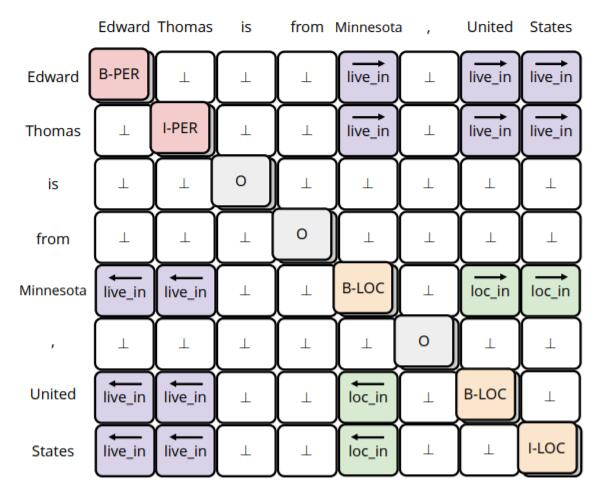• 在主对角线中, 实体被BIO标注法标出, NER被完成.
• 在对角线两侧, 不同的颜色代表不同的关系, 而方向标明了该实体是Subject还是Object, RE被完成.

• 存在特征困惑问题, 即One Feature for Two Task, NER和RE本来是两个不同的任务, 单个Encoder会在学习过程中产生困惑, 不能学习到同时解决NER和RE任务的特征.
• 没有充分使用表结构信息, 大多数的Joint Extraction论文确实构造了表结构, 但是却最后把Table Filling问题转化为一个Sequence Labeling问题去解决.

## TSE

TSE(Table - Sequence Encoders)是我自己给模型起的名字. 相较于后续其他论文起的Table - Sequence, 我还是认为简写更好.

• NER: NER被建模为一个序列标注问题, 其Label $\boldsymbol{y}^{\text {NER}}$ 为标准的BIO标注.
• RE: RE被建模为一个填表问题, 对于给出的句子$\boldsymbol{x}=\left[x_{i}\right]_{1 \leq i \leq N}$, 在标注表中标注出$\boldsymbol{y}^{\mathrm{RE}}=\left[y_{i, j}^{\mathrm{RE}}\right]_{1 \leq i, j \leq N}$. 实体$x_{i^{b}}, \ldots, x_{i^{e}}$ 与实体$x_{j^{b}}, . ., x_{j^{e}}$ 之间的关系定义为$y_{i, j}^{\mathrm{RE}}=\overrightarrow{r}$, 当两实体调换位置时, 记为$y_{j, i}^{\mathrm{RE}}=\overleftarrow{r}$. $\perp$ 代表不存在关系.

TSE主要由三个部分组成: 基础表示Text Embedder, 表表示Table Encoder, 序列表示Sequence Encoder: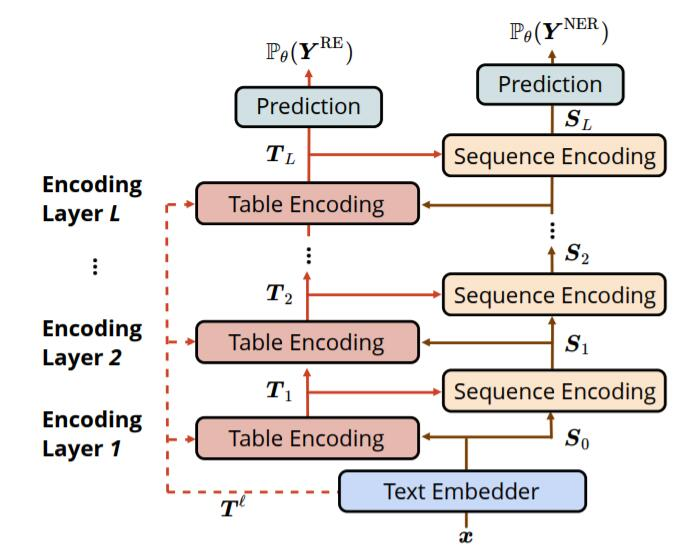### Text Embedder

$$\boldsymbol{S}_{0}=\operatorname{Linear}\left(\left[\boldsymbol{x}^{c} ; \boldsymbol{x}^{w} ; \boldsymbol{x}^{\ell}\right]\right)$$

### Table Encoder

Table Encoder专门学习表示.

$$X_{l, i, j}=\operatorname{ReLU}\left(\operatorname{Linear}\left(\left[S_{l-1, i} ; S_{l-1, j}\right]\right)\right)$$

$$T_{l, i, j}=\operatorname{GRU}\left(X_{l, i, j}, T_{l-1, i, j}, T_{l, i-1, j}, T_{l, i, j-1}\right)$$

$\operatorname{GRU}$ 跨越了三个维度, 除了表的行$i$, 列$j$, 还有跨越不同Table Encoder层的$l$.

\begin{aligned} &T_{l, i, j}^{(a)}=\operatorname{GRU}^{(a)}\left(X_{l, i, j}, T_{l-1, i, j}^{(a)}, T_{l, i-1, j}^{(a)}, T_{l, i, j-1}^{(a)}\right) \\ &T_{l, i, j}^{(c)}=\operatorname{GRU}^{(c)}\left(X_{l, i, j}, T_{l-1, i, j}^{(c)}, T_{l, i+1, j}^{(c)}, T_{l, i, j+1}^{(c)}\right) \\ &T_{l, i, j}=\left[T_{l, i, j}^{(a)} ; T_{l, i, j}^{(c)}\right] \end{aligned}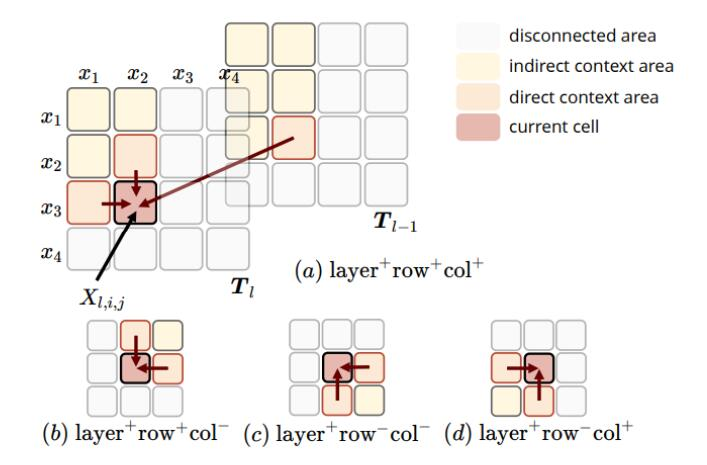### Sequence Encoder

Sequence Encoder专门学习序列表示.

$$f\left(Q_{i}, K_{j}\right)=U \cdot g\left(Q_{i}, K_{j}\right)$$

$f$ 能返回$Q_i$ 下$V_i$ 的权重. 其中$U$ 为可学习参数, $g$ 为计算$Q, K$ 间相关性的函数.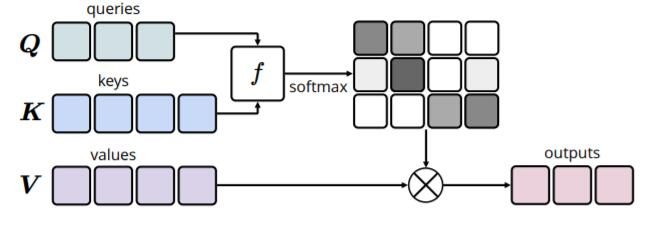$$f\left(Q_{i}, K_{j}\right)=U \cdot T_{l, i, j}$$

1. 不用算$g(\cdot)$, 直接从Table Encoder里拿$\boldsymbol{T}_l$ 就行.
2. 因为$\boldsymbol{T}_l$ 是表内上下文相关的, 所以更能捕捉词间不同相关性.
3. 使得Table Encoder能参与到Sequence Encoder的学习过程中, 从而提升两个Encoder间的交互性.

\begin{aligned} \tilde{\boldsymbol{S}}_{l} &=\operatorname{LayerNorm}\left(\boldsymbol{S}_{l-1}+\operatorname{SelfAttn}\left(\boldsymbol{S}_{l-1}\right)\right) \\ \boldsymbol{S}_{l} &=\operatorname{LayerNorm}\left(\tilde{\boldsymbol{S}}_{l}+\operatorname{FFNN}\left(\tilde{\boldsymbol{S}}_{l}\right)\right) \end{aligned}### Exploit Pre - trained Attention Weights

$$\displaylines{X_{l, i, j}=\operatorname{ReLU}\left(\operatorname{Linear}\left(\left[S_{l-1, i} ; S_{l-1, j}\right]\right)\right) \\ \downarrow \\ X_{l, i, j}=\operatorname{ReLU}\left(\operatorname{Linear}\left(\left[S_{l-1, i} ; S_{l-1, j} ; T_{i, j}^{\ell}\right]\right)\right)}$$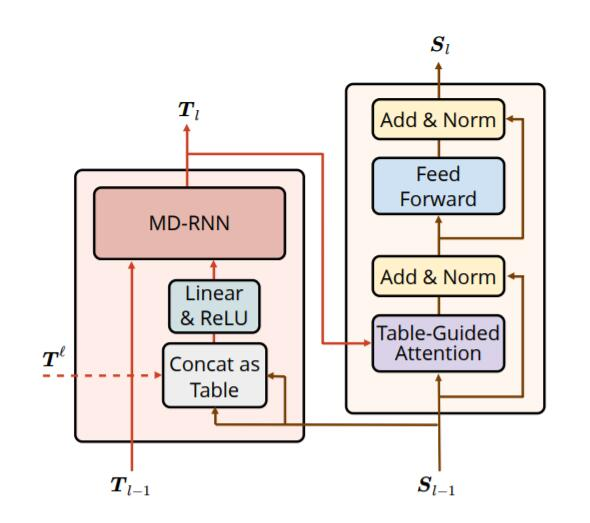1. 由Sequence Encoding和预训练模型的Attention Score一起得到最初的Table Encoding.
2. Table Encoding内部用MD - RNN做表间和跨层的交互.
3. Table信息通过Table - Guided Attention反作用于Sequence Encoder的训练.

### Training and Evaluation

\begin{aligned} P_{\theta}\left(\boldsymbol{Y}^{\mathrm{NER}}\right) &=\operatorname{softmax}\left(\operatorname{Linear}\left(\boldsymbol{S}_{L}\right)\right) \\ P_{\theta}\left(\boldsymbol{Y}^{\mathrm{RE}}\right) &=\operatorname{softmax}\left(\operatorname{Linear}\left(\boldsymbol{T}_{L}\right)\right) \end{aligned}

\begin{aligned} \mathcal{L}^{\mathrm{NER}} &=\sum_{i \in[1, N]}-\log P_{\theta}\left(Y_{i}^{\mathrm{NER}}=y_{i}^{\mathrm{NER}}\right) \\ \mathcal{L}^{\mathrm{RE}} &=\sum_{i, j \in[1, N] ; i \neq j}-\log P_{\theta}\left(Y_{i, j}^{\mathrm{RE}}=y_{i, j}^{\mathrm{RE}}\right) \end{aligned}

$$\underset{e}{\operatorname{argmax}} P_{\theta}\left(Y_{i}^{\mathrm{NER}}=e\right)$$

$$\underset{\vec{r}}{\operatorname{argmax}} \sum_{i \in\left[i^{b}, i^{e}\right], j \in\left[j^{b}, j^{e}\right]} P_{\theta}\left(Y_{i, j}^{\mathrm{RE}}=\overrightarrow{r}\right)+P_{\theta}\left(Y_{j, i}^{\mathrm{RE}}=\overleftarrow{r}\right)$$

$\perp$ 代表不存在关系, 它是不存在方向的.

## Experiments

### Comparison with Other Models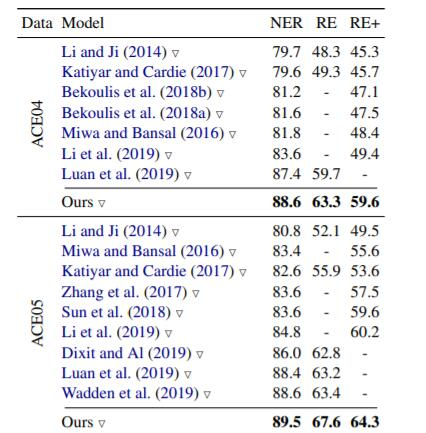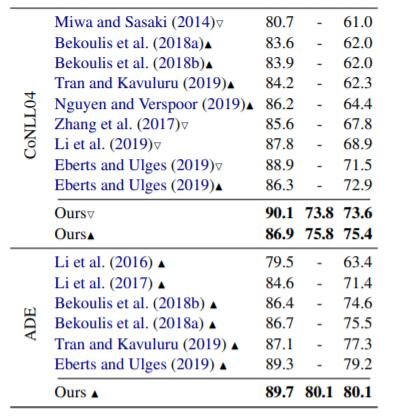RE + 代表实体边界, 以及实体类型, 关系类型, 三者均匹配时才算正确, 比传统的RE任务要更加严格, 还包含了实体类型.

### Comparison of Pre - trained Models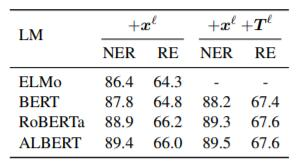$+\boldsymbol{x}^\ell$ 代表使用了上下文相关的Word Embedding, $+\boldsymbol{T}^\ell$ 代表使用了Attention Weights.

### Ablation Study

#### Bidirectional Interaction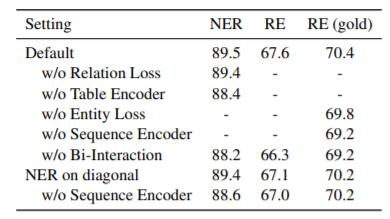RE(gold) 代表在做RE时候直接使用Golden Entity.

• 不使用NER Loss或者不使用RE Loss, 都会降低性能, NER和RE彼此间确实存在关联, 且有相互促进作用.
• 不使用Table Encoder或不使用Sequence Encoder都会对性能产生负面影响, 如果切断两个Encoder之间的交互, 效果会更差.
• 如果直接把Table Representation的主对角线用来做NER任务, 也就是把NER和RE统一在一个空间中解决, 效果也不错, 但达不到作者的设置. 作者认为这是因为NER和RE仍然存在潜在差异, 不能直接用RE任务的Feature来做NER. 在该基础上, 继续去掉Sequence Encoder, 效果会变差, 这表明了Sequence信息对NER的作用.

#### Encoding Layers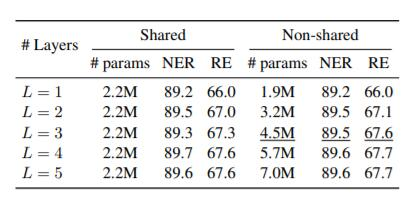#### Settings of MD - RNN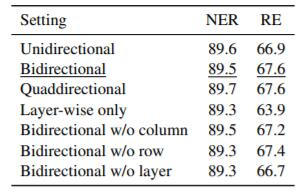### Attention Visualization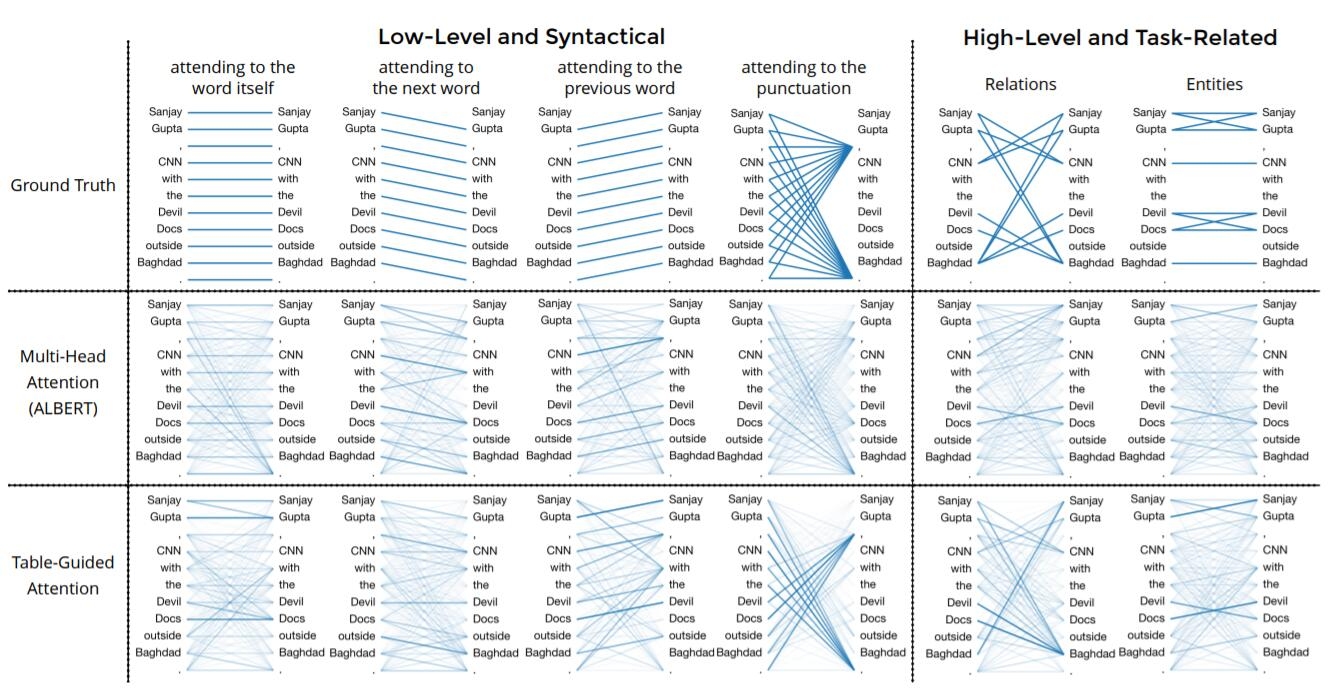### Probing Intermediate States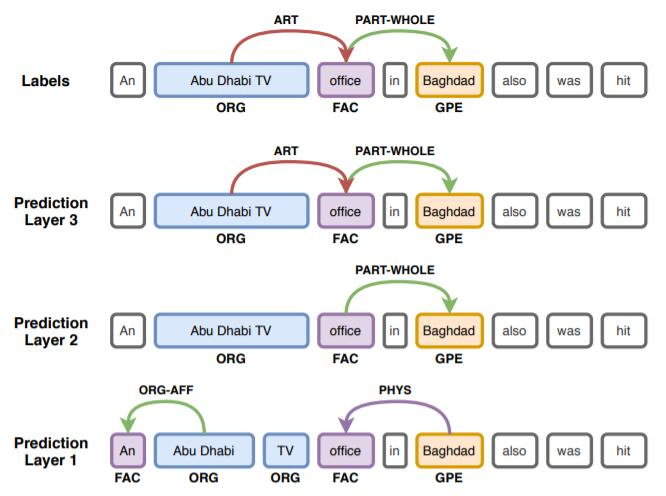Probing在这里起作用的原因是作者引入了跨Encoder层信息.

## Summary

上一篇PURE: A Frustratingly Easy Approach for Entity and Relation Extraction
A Frustratingly Easy Approach for Entity and Relation Extraction本文是论文A Frustratingly Easy Approach for Entity and Relati
2021-12-01

目录